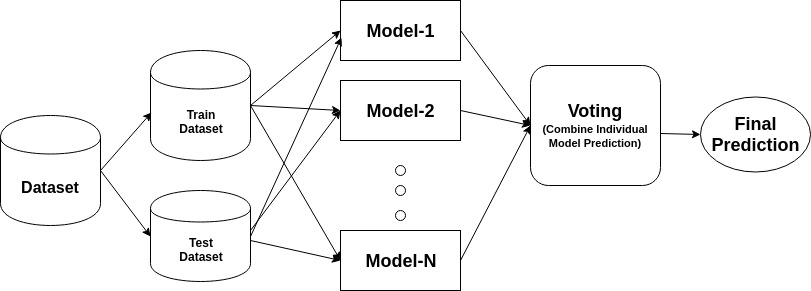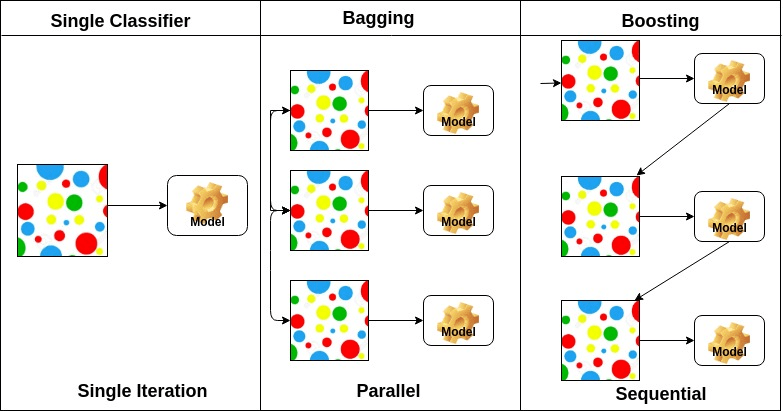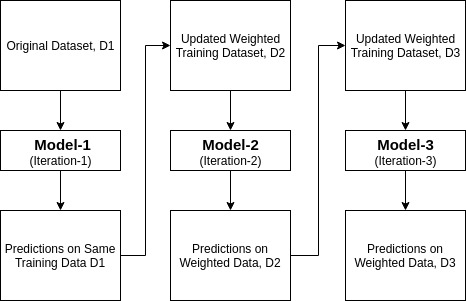Tutorials
python

Understand the ensemble approach, working of the AdaBoost algorithm and learn AdaBoost model building in Python.

In recent years, boosting algorithms gained massive popularity in data science or machine learning competitions. Most of the winners of these competitions use boosting algorithms to achieve high accuracy. These Data science competitions provide the global platform for learning, exploring and providing solutions for various business and government problems. Boosting algorithms combine multiple low accuracy(or weak) models to create a high accuracy(or strong) models. It can be utilized in various domains such as credit, insurance, marketing, and sales. Boosting algorithms such as AdaBoost, Gradient Boosting, and XGBoost are widely used machine learning algorithm to win the data science competitions. In this tutorial, you are going to learn the AdaBoost ensemble boosting algorithm, and the following topics will be covered:

• Ensemble Machine Learning Approach
• Bagging
• Boosting
• stacking
• How does the AdaBoost algorithm work?
• Building Model in Python
• Pros and cons
• Conclusion

Ensemble Machine Learning Approach

An ensemble is a composite model, combines a series of low performing classifiers with the aim of creating an improved classifier. Here, individual classifier vote and final prediction label returned that performs majority voting. Ensembles offer more accuracy than individual or base classifier. Ensemble methods can parallelize by allocating each base learner to different-different machines. Finally, you can say Ensemble learning methods are meta-algorithms that combine several machine learning methods into a single predictive model to increase performance. Ensemble methods can decrease variance using bagging approach, bias using a boosting approach, or improve predictions using stacking approach.1. Bagging stands for bootstrap aggregation. It combines multiple learners in a way to reduce the variance of estimates. For example, random forest trains M Decision Tree, you can train M different trees on different random subsets of the data and perform voting for final prediction. Bagging ensembles methods are Random Forest and Extra Trees.

2. Boosting algorithms are a set of the low accurate classifier to create a highly accurate classifier. Low accuracy classifier (or weak classifier) offers the accuracy better than the flipping of a coin. Highly accurate classifier( or strong classifier) offer error rate close to 0. Boosting algorithm can track the model who failed the accurate prediction. Boosting algorithms are less affected by the overfitting problem. The following three algorithms have gained massive popularity in data science competitions.

• XGBoost
3. Stacking(or stacked generalization) is an ensemble learning technique that combines multiple base classification models predictions into a new data set. This new data are treated as the input data for another classifier. This classifier employed to solve this problem. Stacking is often referred to as blending.On the basis of the arrangement of base learners, ensemble methods can be divided into two groups: In parallel ensemble methods, base learners are generated in parallel for example. Random Forest. In sequential ensemble methods, base learners are generated sequentially for example AdaBoost.

On the basis of the type of base learners, ensemble methods can be divided into two groups: homogenous ensemble method uses the same type of base learner in each iteration. heterogeneous ensemble method uses the different type of base learner in each iteration.

Ada-boost or Adaptive Boosting is one of ensemble boosting classifier proposed by Yoav Freund and Robert Schapire in 1996. It combines multiple classifiers to increase the accuracy of classifiers. AdaBoost is an iterative ensemble method. AdaBoost classifier builds a strong classifier by combining multiple poorly performing classifiers so that you will get high accuracy strong classifier. The basic concept behind Adaboost is to set the weights of classifiers and training the data sample in each iteration such that it ensures the accurate predictions of unusual observations. Any machine learning algorithm can be used as base classifier if it accepts weights on the training set. Adaboost should meet two conditions:

1. The classifier should be trained interactively on various weighed training examples.
2. In each iteration, it tries to provide an excellent fit for these examples by minimizing training error.

How does the AdaBoost algorithm work?

It works in the following steps:

1. Initially, Adaboost selects a training subset randomly.
2. It iteratively trains the AdaBoost machine learning model by selecting the training set based on the accurate prediction of the last training.
3. It assigns the higher weight to wrong classified observations so that in the next iteration these observations will get the high probability for classification.
4. Also, It assigns the weight to the trained classifier in each iteration according to the accuracy of the classifier. The more accurate classifier will get high weight.
5. This process iterate until the complete training data fits without any error or until reached to the specified maximum number of estimators.
6. To classify, perform a "vote" across all of the learning algorithms you built.Building Model in Python

Importing Required Libraries

Let's first load the required libraries.

from sklearn import datasets
# Import train_test_split function
from sklearn.model_selection import train_test_split
#Import scikit-learn metrics module for accuracy calculation
from sklearn import metrics

In the model the building part, you can use the IRIS dataset, which is a very famous multi-class classification problem. This dataset comprises 4 features (sepal length, sepal width, petal length, petal width) and a target (the type of flower). This data has three types of flower classes: Setosa, Versicolour, and Virginica. The dataset is available in the scikit-learn library, or you can also download it from the UCI Machine Learning Library.

X = iris.data
y = iris.target

Split dataset

To understand model performance, dividing the dataset into a training set and a test set is a good strategy.

Let's split dataset by using function train_test_split(). you need to pass 3 parameters features, target, and test_set size.

# Split dataset into training set and test set
X_train, X_test, y_train, y_test = train_test_split(X, y, test_size=0.3) # 70% training and 30% test

Let's create the AdaBoost Model using Scikit-learn. AdaBoost uses Decision Tree Classifier as default Classifier.

learning_rate=1)
model = abc.fit(X_train, y_train)

#Predict the response for test dataset
y_pred = model.predict(X_test)

"The most important parameters are base_estimator, n_estimators, and learning_rate." (Adaboost Classifier, Chris Albon)

• base_estimator: It is a weak learner used to train the model. It uses DecisionTreeClassifier as default weak learner for training purpose. You can also specify different machine learning algorithms.
• n_estimators: Number of weak learners to train iteratively.
• learning_rate: It contributes to the weights of weak learners. It uses 1 as a default value.

Evaluate Model

Let's estimate, how accurately the classifier or model can predict the type of cultivars.

Accuracy can be computed by comparing actual test set values and predicted values.

# Model Accuracy, how often is the classifier correct?
print("Accuracy:",metrics.accuracy_score(y_test, y_pred))
Accuracy: 0.8888888888888888

Well, you got an accuracy of 88.88%, considered as good accuracy.

For further evaluation, you can also create a model using different Base Estimators.

Using Different Base Learners

I have used SVC as a base estimator. You can use any ML learner as base estimator if it accepts sample weight such as Decision Tree, Support Vector Classifier.

# Import Support Vector Classifier
from sklearn.svm import SVC
#Import scikit-learn metrics module for accuracy calculation
from sklearn import metrics
svc=SVC(probability=True, kernel='linear')

model = abc.fit(X_train, y_train)

#Predict the response for test dataset
y_pred = model.predict(X_test)

# Model Accuracy, how often is the classifier correct?
print("Accuracy:",metrics.accuracy_score(y_test, y_pred))
Accuracy: 0.9555555555555556

Well, you got a classification rate of 95.55%, considered as good accuracy.

In this case, SVC Base Estimator is getting better accuracy then Decision tree Base Estimator.

Pros

AdaBoost is easy to implement. It iteratively corrects the mistakes of the weak classifier and improves accuracy by combining weak learners. You can use many base classifiers with AdaBoost. AdaBoost is not prone to overfitting. This can be found out via experiment results, but there is no concrete reason available.

Cons

AdaBoost is sensitive to noise data. It is highly affected by outliers because it tries to fit each point perfectly. AdaBoost is slower compared to XGBoost.

Conclusion

Congratulations, you have made it to the end of this tutorial!

In this tutorial, you have learned the Ensemble Machine Learning Approaches, AdaBoost algorithm, it's working, model building and evaluation using Python Scikit-learn package. Also, discussed its pros and cons.

I look forward to hearing any feedback or questions. You can ask a question by leaving a comment, and I will try my best to answer it.